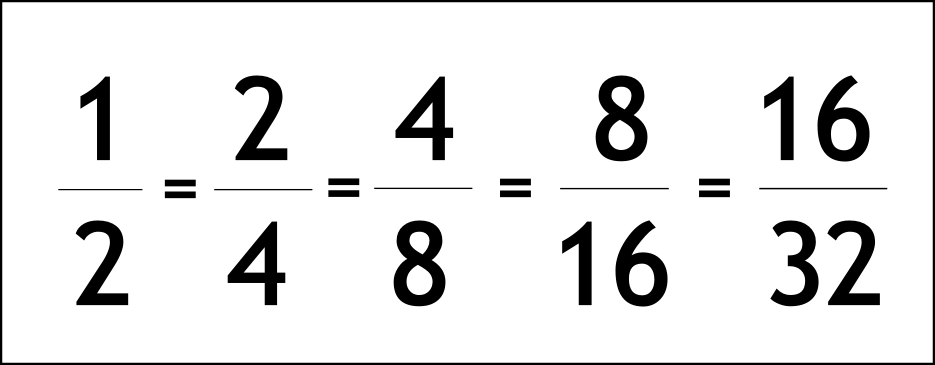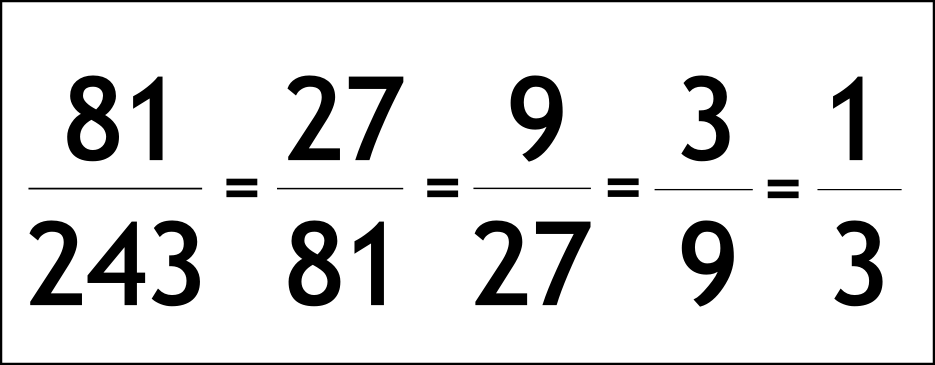The Singing Hedgehog Guide to:
Equivalent Fractions 1

When changing fractions we have to remember the rule:

DO THE SAME TO THE TOP,
AS YOU DO TO THE BOTTOM

Equivlalent fractions are all equal, the same value.
Here are two examples.

If we start with a half, we can double top and bottom to get this series:Division works too, here is a set of dividing top and bottom by three:-

next page

fractions index